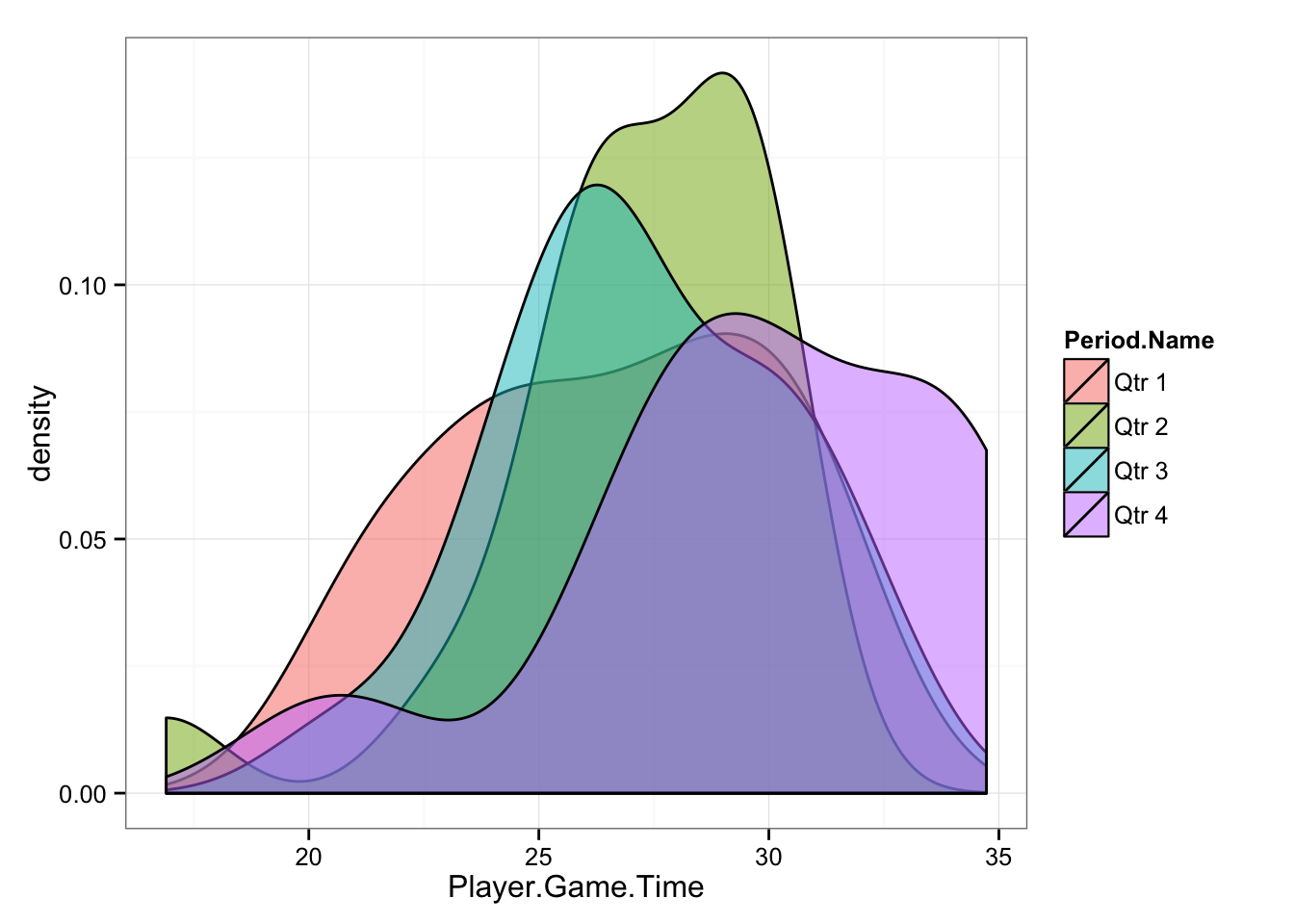## Introduction

This data set is part of the #UCSIA15 course by Keith Lyons. The Google docs shared by Keith could be found here. More infor about the data set can be found here

I have downloaded the sheet, converted it to .CSV and removed #REF! from rows 81-85. This will cause missing values in the data set (NAs) that need to be dealt with appropriately. More on this later.

Anyway, here is the data set:

``````suppressPackageStartupMessages(library(googleVis))

The dimensions of the data are the 84x200. Since there are more features (columns) than observations, it makes this data set highly dimensional, which is problematic for some analysis.

The data represents GPS analysis of one AFL game, split into quarters. The scores by quarter in the game were:

33 v 22 (Q1)

33 v 22 (Q2)

33 v 26 (Q3)

33 v 37 (Q4)

The data doesn’t contains any ID of the players, so it is impossible to perform any type of single-subject analysis, but rather perform it on the team level.

The goal of the analysis is try to find if the GPS data can explain quarters scores.

## Data munging

Before data can be used it needs to be cleaned and prepared, and usually that is the longest and toughest part of data analysis.

As said previously some of the rows contains missing values. Lets see what columns contain missing values

``````nacols <- function(df) {
colnames(df)[unlist(lapply(df, function(x) any(is.na(x))))]
}

nacols(data)``````
``````##  "IMA.High.CoD.min" "mins"             "mins.coverted."
##  "IMA.High.CoD.R.."``````

And let’s see what quarters contain the most missing data

``table(data\$Period.Name, rowSums(is.na(data)))``
``````##
##          0  4
##   Qtr 1 21  0
##   Qtr 2 21  0
##   Qtr 3 21  0
##   Qtr 4 16  5``````

Appartently, Quarter 4 contains all missing data. Removing these players (rows) might remove interesting data from Quarter four. One option is to imputate missing values, either using averages for the column, or using KNN algorythim. For this analysis I will remove the columns containing missing data

``````# Remove the columns with missing data
data <- data[!(names(data) %in% nacols(data))]``````

Another issue we need to deal with is the format for time for some of the columns, since they use “00:10:13” and we want to convert it to seconds.

``````suppressPackageStartupMessages(library(lubridate))
suppressPackageStartupMessages(library(dplyr))

# Convert to factors the colums we are certain it doesn't contain time
data\$Position <- factor(data\$Position)
data\$Period.Name <- factor(data\$Period.Name)

# Create a function that return a class of the column
allClass <- function(x) {unlist(lapply(unclass(x),class))}

# Get the column classes
column.classes <- allClass(data)

# What columns contain time as character
time.columns.positions <- which(column.classes == "character")

# Select those columns
data.time <- data %>% select(time.columns.positions)

# Convert to seconds
data.time <- sapply(data.time, function(x) {period_to_seconds(hms(x))} )

# Put them back in the original data frame
data[colnames(data.time)] <- data.time``````

Since not all players played the full quarters, the GPS metrics need to be normalized per playing time. There is one problems with that - some metrics are already normalized (e.g. Distance / min), some shouldn’t be normalized (e.g. % in Zone 5, max velocity). This is why it is important to have domain knowledge of the features.

Since there are ~200 features it is hard to go over each (especially beause their names changed when importing with the removal of spaces and special characters) to see which one needs to be normalized.

What we can do instead is to remove plyers who didn’t play enough in the game. This is VERY IMPORTANT since these modifications and assumptions can affect the results of the analysis.

Let’s see the distribution of playing time across quarters

``````aggregate(Player.Game.Time~Period.Name, data,
function(x){c(MEAN = mean(x), SD = sd(x), N = length(x))})``````
``````##   Period.Name Player.Game.Time.MEAN Player.Game.Time.SD Player.Game.Time.N
## 1       Qtr 1             26.568571            3.484331          21.000000
## 2       Qtr 2             27.078095            3.138332          21.000000
## 3       Qtr 3             27.249524            3.073313          21.000000
## 4       Qtr 4             29.755714            4.115769          21.000000``````
``````# The distribution on play time across quarters
library(ggplot2)
gg <- ggplot(data, aes(Player.Game.Time, fill = Period.Name))
gg <- gg + geom_density(alpha = 0.5)
gg <- gg + theme_bw()
gg``````If we use data as it is, without normalization for time, then the effects might be related to longer fourth quarter. But let’s see if the differences are statistically significant (I haven’t check the normality of the data) using ANOVA

``summary(aov(Player.Game.Time ~ Period.Name, data))``
``````##             Df Sum Sq Mean Sq F value Pr(>F)
## Period.Name  3  127.9   42.63   3.525 0.0186 *
## Residuals   80  967.5   12.09
## ---
## Signif. codes:  0 '***' 0.001 '**' 0.01 '*' 0.05 '.' 0.1 ' ' 1``````

We can see from the ANOVA that we cannot use quarters data as they are since that will affect the effects. We need to normalize the data or create cut-off for play time to make quarters equal. Since I cannot normalize the data because I don’t know which columns need to be normalized (divided by play time) and it is ~200 of them, I will need to remove some of the players (observations) to make quarters more equal in play time.

It is very important to notice that this procedure might/will affect the analysis, but without the list of features (columns) that needs to be normalized I cannot procees with the analysis.

``````# Keep observations with less than 32 minutes of play
data.mod <- data %>% filter(Player.Game.Time < 32)

# Check the differences between quarters
aggregate(Player.Game.Time~Period.Name, data.mod,
function(x){c(MEAN = mean(x), SD = sd(x), N = length(x))})``````
``````##   Period.Name Player.Game.Time.MEAN Player.Game.Time.SD Player.Game.Time.N
## 1       Qtr 1             26.568571            3.484331          21.000000
## 2       Qtr 2             27.078095            3.138332          21.000000
## 3       Qtr 3             26.714737            2.706460          19.000000
## 4       Qtr 4             27.675714            3.409556          14.000000``````
``````# plot
gg <- ggplot(data.mod, aes(Player.Game.Time, fill = Period.Name))
gg <- gg + geom_density(alpha = 0.5)
gg <- gg + theme_bw()
gg``````